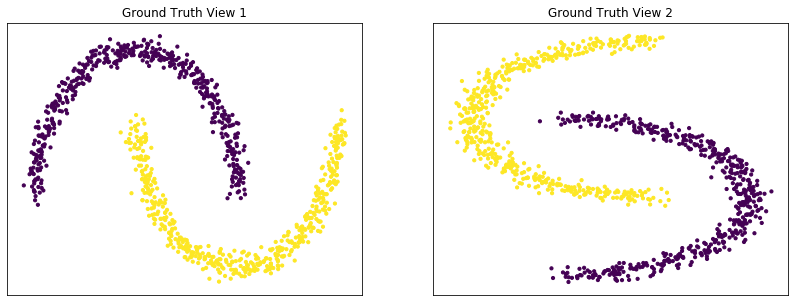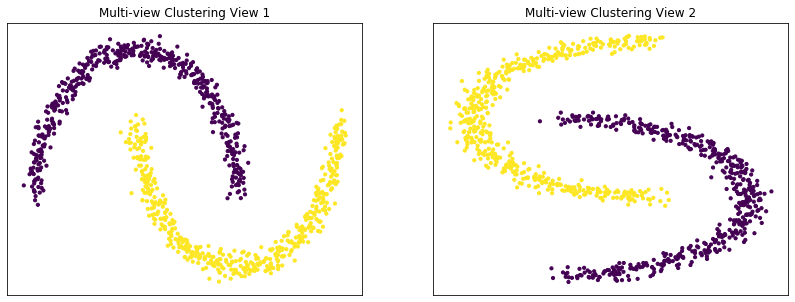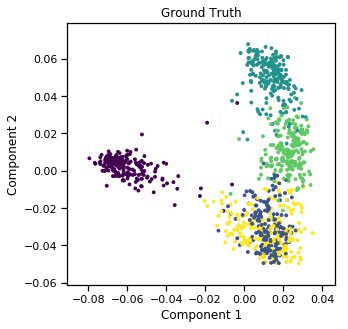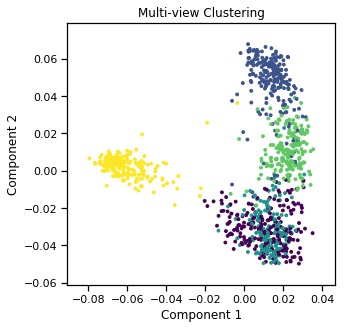# Multi-view Spectral Clustering¶

:

from mvlearn.datasets import load_UCImultifeature
from mvlearn.cluster import MultiviewSpectralClustering
from mvlearn.plotting import quick_visualize
import numpy as np
from sklearn.cluster import SpectralClustering
from sklearn.metrics import normalized_mutual_info_score as nmi_score
from sklearn.datasets import make_moons
import matplotlib.pyplot as plt
import scipy
import warnings

warnings.simplefilter('ignore') # Ignore warnings
%matplotlib inline
RANDOM_SEED=10


## Creating a function to display data and the results of clustering¶

The following function plots both views of data given a dataset and corresponding labels.

:

def display_plots(pre_title, data, labels):

# plot the views
plt.figure()
fig, ax = plt.subplots(1,2, figsize=(14,5))
dot_size=10
ax.scatter(data[:, 0], data[:, 1],c=labels,s=dot_size)
ax.set_title(pre_title + ' View 1')
ax.axes.get_xaxis().set_visible(False)
ax.axes.get_yaxis().set_visible(False)

ax.scatter(data[:, 0], data[:, 1],c=labels,s=dot_size)
ax.set_title(pre_title + ' View 2')
ax.axes.get_xaxis().set_visible(False)
ax.axes.get_yaxis().set_visible(False)

plt.show()


### Performance on moons dataset¶

For this example, we use the sklearn make_moons function to make two interleaving half circles in two views. We then use spectral clustering to separate the two views. As we can see below, multi-view spectral clustering is capable of effectively clustering non-convex cluster shapes, similarly to its single-view analog.

:

# A function to generate the moons data
def create_moons(seed, num_per_class=500):

np.random.seed(seed)
data = []
labels = []

for view in range(2):
v_dat, v_labs = make_moons(num_per_class*2,
random_state=seed + view, noise=0.05, shuffle=False)
if view == 1:
v_dat = v_dat[:, ::-1]

data.append(v_dat)
for ind in range(len(data)):
labels.append(ind * np.ones(num_per_class,))
labels = np.concatenate(labels)

return data, labels

:

# Generating the data
m_data, labels = create_moons(RANDOM_SEED)
n_class = 2

#################Single-view spectral clustering#####################
# Cluster each view separately
s_spectral = SpectralClustering(n_clusters=n_class,
affinity='nearest_neighbors', random_state=RANDOM_SEED, n_init=100)
s_clusters_v1 = s_spectral.fit_predict(m_data)
s_clusters_v2 = s_spectral.fit_predict(m_data)

# Concatenate the multiple views into a single view
s_data = np.hstack(m_data)
s_clusters = s_spectral.fit_predict(s_data)

# Compute nmi between true class labels and single-view cluster labels
s_nmi_v1 = nmi_score(labels, s_clusters_v1)
s_nmi_v2 = nmi_score(labels, s_clusters_v2)
s_nmi = nmi_score(labels, s_clusters)
print('Single-view View 1 NMI Score: {0:.3f}\n'.format(s_nmi_v1))
print('Single-view View 2 NMI Score: {0:.3f}\n'.format(s_nmi_v2))
print('Single-view Concatenated NMI Score: {0:.3f}\n'.format(s_nmi))

#################Multi-view spectral clustering######################

# Use the MultiviewSpectralClustering instance to cluster the data
m_spectral = MultiviewSpectralClustering(n_clusters=n_class,
affinity='nearest_neighbors', max_iter=12, random_state=RANDOM_SEED, n_init=100)
m_clusters = m_spectral.fit_predict(m_data)

# Compute nmi between true class labels and multi-view cluster labels
m_nmi = nmi_score(labels, m_clusters)
print('Multi-view NMI Score: {0:.3f}\n'.format(m_nmi))

Single-view View 1 NMI Score: 1.000

Single-view View 2 NMI Score: 1.000

Single-view Concatenated NMI Score: 1.000

Multi-view NMI Score: 1.000



## Plots of clusters produced by multi-view spectral clustering and the true clusters¶

We will display the clustering results of the Multi-view spectral clustering algorithm below, along with the true class labels.

:

display_plots('Ground Truth' , m_data, labels)
display_plots('Multi-view Clustering' , m_data, m_clusters)

<Figure size 432x288 with 0 Axes><Figure size 432x288 with 0 Axes>### Performance on the UCI Digits Multiple Features data set with 2 views¶

Here we will compare the performance of the Multi-view and Single-view versions of spectral clustering. We will evaluate the purity of the resulting clusters from each algorithm with respect to the class labels using the normalized mutual information metric.

As we can see, Multi-view clustering produces clusters with higher purity compared to those produced by Single-view clustering for all 3 input types.

:

# Load dataset along with labels for digits 0 through 4
n_class = 5
m_data, labels = load_UCImultifeature(select_labeled = list(range(n_class)))

:

#################Single-view spectral clustering#####################
# Cluster each view separately
s_spectral = SpectralClustering(n_clusters=n_class, random_state=RANDOM_SEED, n_init=100)

for i in range(len(m_data)):
s_clusters = s_spectral.fit_predict(m_data[i])
s_nmi = nmi_score(labels, s_clusters, average_method='arithmetic')
print('Single-view View {0:d} NMI Score: {1:.3f}\n'.format(i + 1, s_nmi))

# Concatenate the multiple views into a single view and produce clusters
s_data = np.hstack(m_data)
s_clusters = s_spectral.fit_predict(s_data)

s_nmi = nmi_score(labels, s_clusters)
print('Single-view Concatenated NMI Score: {0:.3f}\n'.format(s_nmi))

#################Multi-view spectral clustering######################

# Use the MultiviewSpectralClustering instance to cluster the data
m_spectral1 = MultiviewSpectralClustering(n_clusters=n_class,
random_state=RANDOM_SEED, n_init=100)
m_clusters1 = m_spectral1.fit_predict(m_data)

# Compute nmi between true class labels and multi-view cluster labels
m_nmi1 = nmi_score(labels, m_clusters1)
print('Multi-view NMI Score: {0:.3f}\n'.format(m_nmi1))

Single-view View 1 NMI Score: 0.620

Single-view View 2 NMI Score: 0.007

Single-view View 3 NMI Score: 0.004

Single-view View 4 NMI Score: -0.000

Single-view View 5 NMI Score: 0.007

Single-view View 6 NMI Score: 0.010

Single-view Concatenated NMI Score: 0.008

Multi-view NMI Score: 0.881



## Plots of clusters produced by multi-view spectral clustering and the true clusters¶

We will display the clustering results of the Multi-view spectral clustering algorithm below, along with the true class labels.

:

quick_visualize(m_data, labels=labels, title='Ground Truth', scatter_kwargs={'s':8})
quick_visualize(m_data, labels=m_clusters1, title='Multi-view Clustering', scatter_kwargs={'s':8})